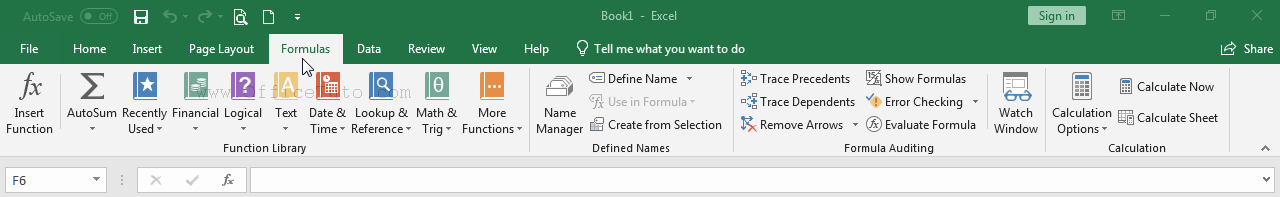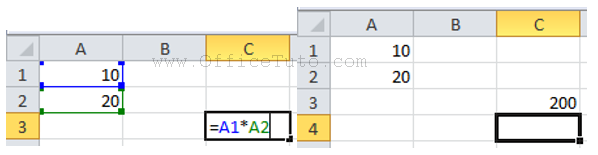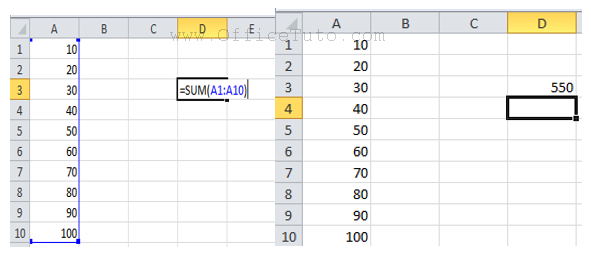Excel formulas

Microsoft Excel is, at the same time, a spreadsheet program, a data visualization tool, and a data analysis tool. It can be all of that thanks to an essential and important feature that is formulas and functions.

In the following, we will present an overview of Microsoft Excel formulas, how to create them, how to use them along with functions, and how to copy them to an entire column or row.

– Introduction

Excel Formulas are comparable to real-life mathematics formulas. They have operators and operands, and they allow to perform calculations, but they offer more complexity with the help of functions and conditions.

An operand in a basic Excel formula is a cell reference. A cell in Excel is the intersection of a row and a column. The cells are named by certain naming conventions and they are used to define a range of data in the formulas. Moreover, the great advantage that Excel has to offer is its wide range of formulas. They are not limited to a certain number. But, users can design custom formulas to carry out their operations.Furthermore, Excel has all popular formulas predefined. Starting from simple formulas like sum to formulas like calculating averages, Excel has all of them built-in.

– How to create a formula

Each Excel formula starts with an equal to (=) sign. It is followed by the math-like formula. For example: =A1*A2. This formula will multiply the value in cell A1 and value in cell A2 and output the result in the desired cell. But, you are not compelled to use a cell name. You can also try using this function to do simple mathematical operations like =10+20. This will output 30 in the selected cell.Another amazing feature of MS Excel is the function. This makes it easy for everyone to use. If you do not know how to write a formula, don’t worry. Excel functions offer great help to you. All you need to do is to select a range of cells. Click on Formulas tab in the ribbon and select the required function. You are done. The output will show up in the next cell. Such features help the users a lot. Due to these features, the work of many users has become easy. Not to mention, the ease of use of MS office products contributes a lot to its popularity among users.

– How to use a function in Excel without using graphical range selector

It is just simple and short. This is the syntax for it: =FORMULA_NAME(START_CELL_NAME:END_CELL_NAME). For an example, let us consider the sum function. It looks like: =SUM(A1:A10) this will output the summation of values of all the cells from A1 to A10 in the selected cell.Also, Excel makes it easy for users to find the right functions by grouping them into some categories. Excel has function groups like financial, date time, math and trigonometry, statistical, lookup, database, text, logical, information, engineering and many more. This makes it easy for different kinds of organizations to use Excel for their work. Excel is able to become more and more popular among the users due to these advantages.

– How to edit a formula

Sometimes we may want to modify an existing formula. This can be explained in the following steps.

• Select the cell containing the formula you want to edit.
• Click the formula bar to modify the formula. You can also double-click the cell to view and modify the formula directly within the cell.
• A border will appear around any referenced cells.
• When you’re finished, press Enter on your keyboard or click the checkmark in the formula bar.
• The formula will be updated, and the new value will be displayed in the cell.

Furthermore, if you make any accidental or unwanted changes to the formula, you can press Esc key and Undo it.

– How to copy a formula to an entire column or a row

• Enter the formula to a cell.
• Drag the autofill handle to copy the formula to the rows and columns.

Exclusively, the text category of functions is very fun to use. Microsoft has introduced some new functions from Excel 2016. They are CONCAT, TEXTJOIN, IFS, SWITCH and few more. In conjunction with these functions, they also have introduced some forecasting representation functions. These help the users to predict projection values in a timeline.

In datasheet processing software like MS Excel, the formulas play an important role, obviously. But, not all formula and functions are present as built-in from the company. But, the utility of the functions is not limited to the built-in ones. So, Microsoft has provided the support for custom functions using Visual BASIC. This makes it easier for some professional users to get more out of the software. Also, Microsoft has a big community support for MS Excel. This makes Excel the best datasheet processing software.

One Reply to “Excel formulas”

1.says:

Like!! Really appreciate you sharing this blog post.Really thank you! Keep writing.